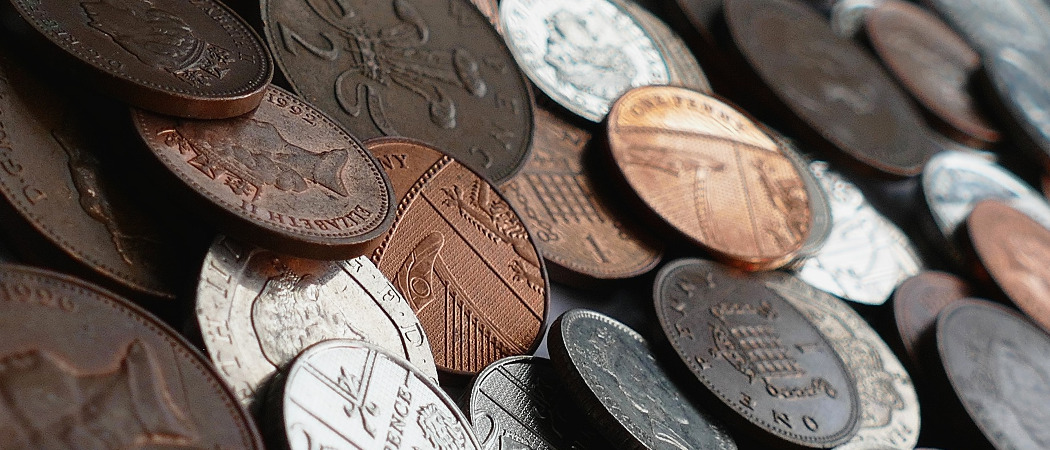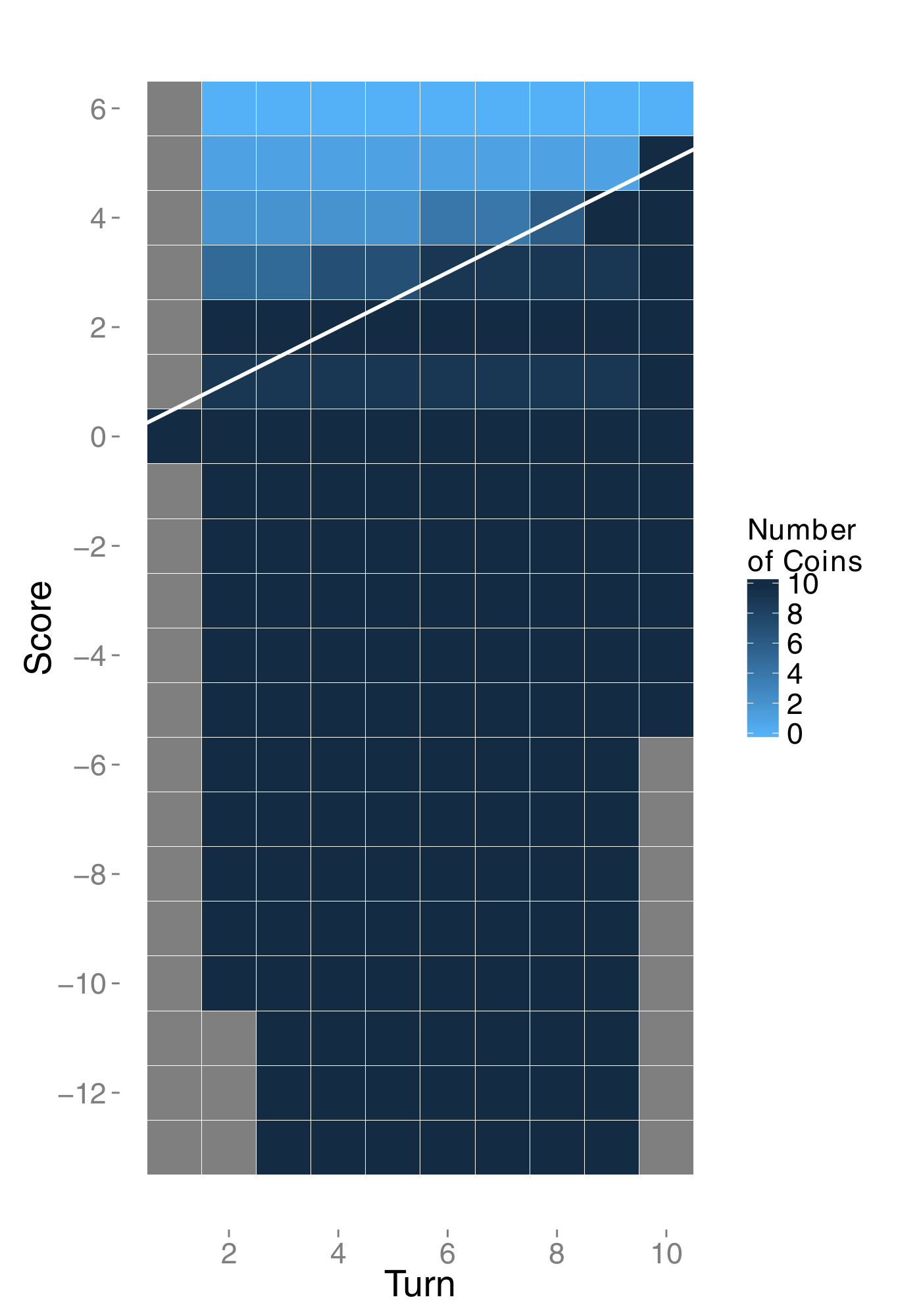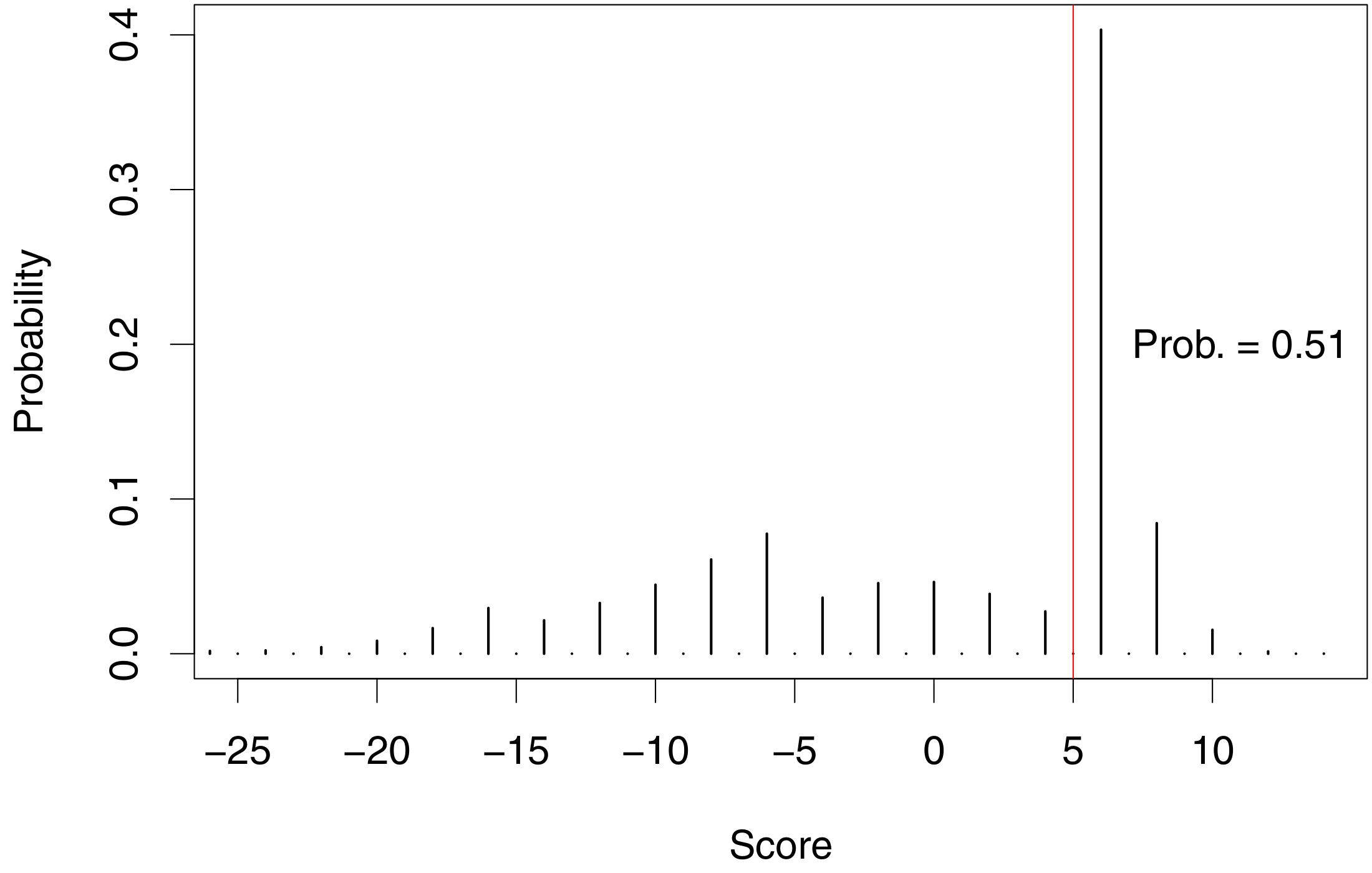# Winning the Chalkdust coin game

How to win a game when your expected score is 0Change from a quid from a self-service machine. Image: public domain.

Go is a two-player strategy game. Players score points for surrounding territory and capturing opponents’ pieces. In 2016, Google challenged the world’s top Go player, Lee Sedol, to a five-game match against their AlphaGo program and won 4-1. The program was successful because it learned to play Go through a machine learning algorithm (specifically deep learning) trained on 30 million moves from games played by human experts (you can read more about AlphaGo here).

But part of AlphaGo’s success is due to its overall strategy: it plays to win rather than to maximise its score. The journal Nature wrote,

The algorithm seems to be holding back its power. Sometimes it plays moves that lose material because it is seeking simply to maximise its probability of reaching winning positions, rather than — as human players tend to do — maximise territorial gains.

In a game where the outcomes are win or lose (or draw) and the margin of victory is irrelevant, your best strategy is to maximise the probability that you win, rather than maximising your expected score. A consequence of this is that if you are leading the game you should be risk averse to hold on to your lead over your opponent, and if you are behind in the game then you should seek risks with large potential payoffs.

This principle will now be demonstrated in an example game that I will call the Chalkdust Coin Game. The game is played over 10 turns. At the beginning of each turn you choose a number of fair coins to flip that turn, up to a maximum of 10 (you can choose to flip none). Suppose you choose to flip $c$ coins. The coins are tossed, you observe $h$ heads, then you add the number of heads minus the number of tails to your score. So your score will increase by
$$h-(c-h)=2h-c.$$
Your opponent’s score increases by $\frac{1}{2}$ each turn, so will be $5$ at the end of $10$ turns. In the Chalkdust Coin Game your expected score is always $0$ no matter how you play. The challenge is to find the strategy that maximises your probability of getting more than $5$ points.

A recursive formula can be created to derive the optimal strategy. Let $w_{t,s}$ denote the probability of winning given that you are on turn $t$ and you have score $s$, and let $c_{t,s}$ denote the number of coins that you should choose on turn $t$ to maximise this probability. Given that

• you are on turn $t$,
• you have score $s$,
• you choose to flip the optimum number of coins $c$ on your next flip,
• and you continue to play optimally afterwards,

then$$w_{t,s}=\sum_{k=0}^{c}\mathbb{P}(k\mathrm{\ heads})w_{t+1,s+2k-c},$$where the number of heads is a binomial random variable with parameters $c,\frac{1}{2}$. This leads to recursive formulas$$w_{t,s} = \max_{c\in\left\{ 0,\dots,10\right\} }\left\{ \sum_{k=0}^{c}\binom{c}{k}\left(\frac{1}{2}\right)^{k}\left(\frac{1}{2}\right)^{c-k}w_{t+1,s+2k-c}\right\} ,$$$$c_{t,s} = \operatorname*{argmax}_{c\in\left\{ 0,\dots,10\right\} }\left\{ \sum_{k=0}^{c}\binom{c}{k}\left(\frac{1}{2}\right)^{k}\left(\frac{1}{2}\right)^{c-k}w_{t+1,s+2k-c}\right\} ,$$with boundary conditions$$w_{11,s}=\begin{cases} 1 & \mathrm{if\ }s>5,\\ \frac{1}{2} & \mathrm{if\ }s=5,\\ 0 & \mathrm{if\ }s<5, \end{cases}$$so 1 point is given for a win, $\frac{1}{2}$ a point is given for a draw and 0 points are given for a loss. This can be solved computationally, and the results are shown below (click to enlarge).Heatmaps showing: (left) the optimal number of coins to use given the turn number and the current score; and (right) the probability of winning, assuming that you play optimally, given the turn number and the current score. The opponent’s score is shown as a white line. Grey squares represent points that are unreachable or where winning is impossible.Distribution of the final scores following the optimal strategy. The expectation of this distribution is 0.

Of course, once your score reaches 6 or higher, you should choose to flip no coins. And if your score is lower than your opponent’s then the best strategy is to gamble heavily. The distribution of the scores obtained in $10^{5}$ simulations of optimally-played games is shown to the right. By coincidence the optimal number of coins in any path taken is even, and so the final score is always an even number (whenever the number of flipped coins $c$ is even then the change in score, $2k-c$, must also be even). The expectation of this distribution must be 0 because the expected value of every coin flip is 0.

When you play optimally you stand a good chance of either winning a narrow victory or losing badly. If you decide beforehand to flip all 100 available coins in the game then your probability of winning is 0.31, but if you play optimally then the probability of winning in this game is 0.51, so the game is actually better than fair.Alex did a statistics PhD at Imperial College London, studying change point detection. He now works as a quantitative analyst at G-Research.
@AlexDBolton    + More articles by Alexander